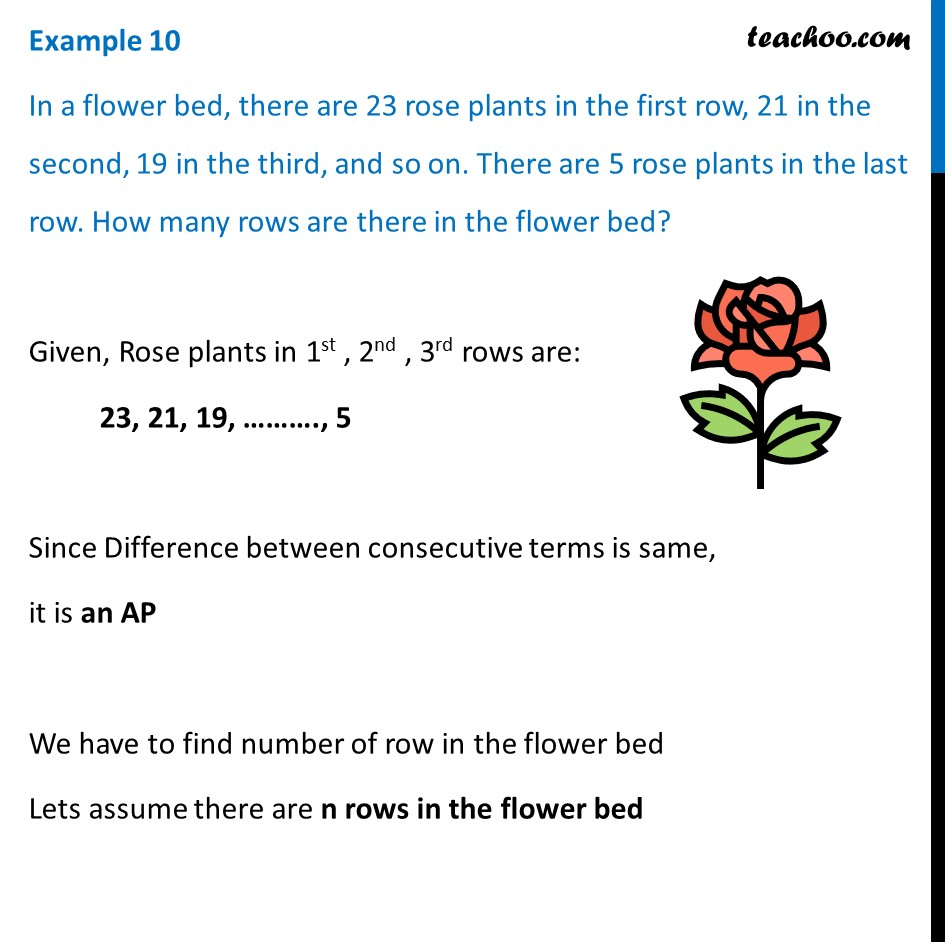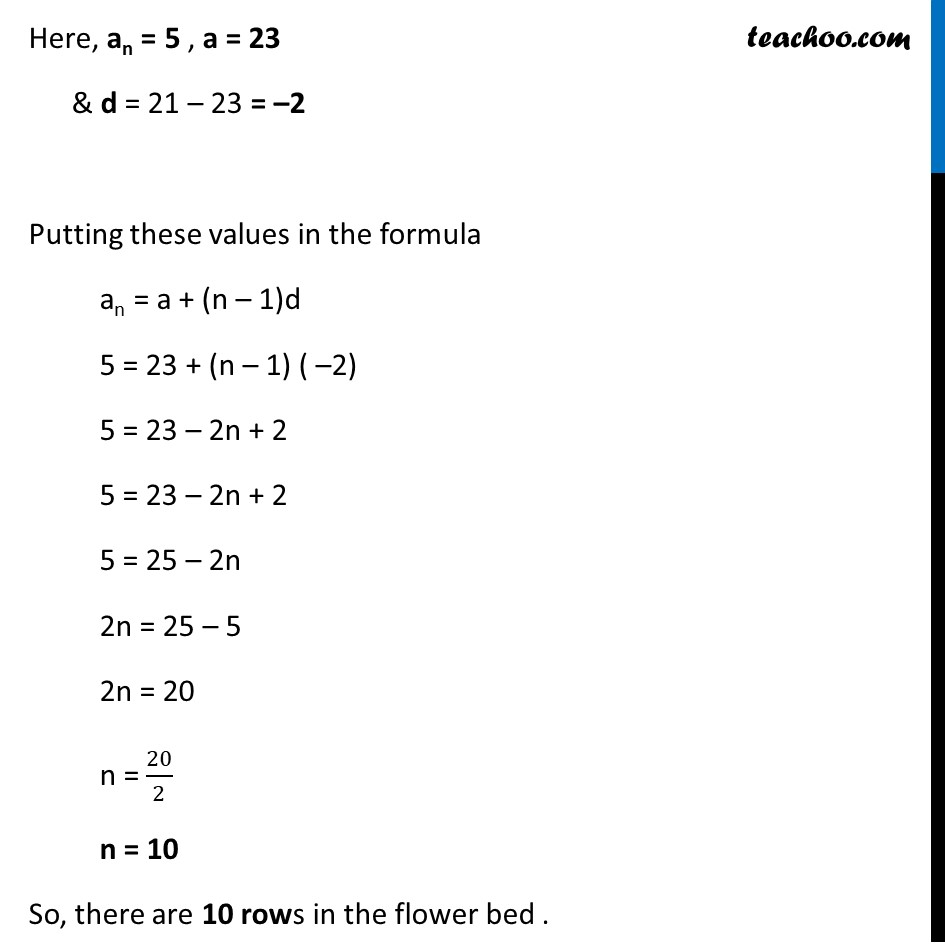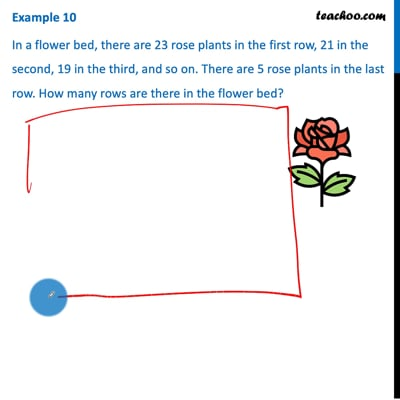Examples

Chapter 5 Class 10 Arithmetic Progressions (Term 2)
Serial order wiseThis video is only available for Teachoo black users

### Transcript

Example 10 In a flower bed, there are 23 rose plants in the first row, 21 in the second, 19 in the third, and so on. There are 5 rose plants in the last row. How many rows are there in the flower bed? Given, Rose plants in 1st , 2nd , 3rd rows are: 23, 21, 19, ………., 5 Since Difference between consecutive terms is same, it is an AP We have to find number of row in the flower bed Lets assume there are n rows in the flower bed Here, an = 5 , a = 23 & d = 21 – 23 = –2 Putting these values in the formula an = a + (n – 1)d 5 = 23 + (n – 1) ( –2) 5 = 23 – 2n + 2 5 = 23 – 2n + 2 5 = 25 – 2n 2n = 25 – 5 2n = 20 n = 20/2 n = 10 So, there are 10 rows in the flower bed .